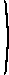# Mathematical methods for economic theory

Martin J. Osborne

## 3.2.2 Quadratic forms: conditions for definiteness

### Definitions

Relevant questions when we use quadratic forms in studying the concavity and convexity of functions of many variables are:
• Under what condition on the matrix A are the values of the quadratic form Q(x) = x'Ax positive for all values of x ≠ 0?
• Under what condition are these values negative for all values of x ≠ 0?
The following terminology is useful.
Definition
Let Q be a quadratic form, and let A be the symmetric matrix that represents it (i.e. Q(x) = x'Ax for all x). Then Q (and the associated matrix A) is
• positive definite if x'Ax > 0 for all x ≠ 0
• negative definite if x'Ax < 0 for all x ≠ 0
• positive semidefinite if x'Ax ≥ 0 for all x
• negative semidefinite if x'Ax ≤ 0 for all x
• indefinite if it is neither positive nor negative semidefinite (i.e. if x'Ax > 0 for some x and x'Ax < 0 for some x).
Example
x2
1
+ x2
2
> 0 if (x1x2) ≠ 0, so this quadratic form is positive definite. More generally, ax2
1
+ cx2
2
is positive definite whenever a > 0 and c > 0
Example
x2
1
+ 2x1x2 + x2
2
may be expressed as (x1 + x2)2, which is nonnegative for all (x1x2). Thus this quadratic form is positive semidefinite. It is not positive definite because (x1 + x2)2 = 0 for (x1x2) = (1,−1) (for example).
Example
x2
1
− x2
2
> 0 for (x1x2) = (1, 0) (for example), and x2
1
− x2
2
< 0 for (x1x2) = (0, 1) (for example). Thus this quadratic form is indefinite.

### Two variables

We can easily derive conditions for the definiteness of any quadratic form in two variables. To make the argument more readable, I change the notation slightly, using x and y for the variables, rather than x1 and x2. Consider the quadratic form
Q(xy) = ax2 + 2bxy + cy2.
If a = 0 then Q(1,0) = 0, so Q is neither positive nor negative definite. So assume that a ≠ 0.

Given a ≠ 0, we have

 Q(x, y) = a[(x + (b/a)y)2 + (c/a − (b/a)2)y2].
Both squares are always nonnegative, and at least one of them is positive unless (x, y) = (0, 0). Thus if a > 0 and c/a − (b/a)2 > 0 then Q(xy) is positive definite. Given a > 0, the second condition is ac > b2. Thus we conclude that if a > 0 and ac > b2 then Q(xy) is positive definite.

Now, we have Q(1, 0) = a and Q(−b/a, 1) = (ac − b2)/a. Thus, if Q(xy) is positive definite then a > 0 and ac > b2.

We conclude that Q(xy) is positive definite if and only if a > 0 and ac > b2.

A similar argument shows that Q(xy) is negative definite if and only if a < 0 and ac > b2.

Note that if a > 0 and ac > b2 then because b2 ≥ 0 for all b, we can conclude that c > 0. Similarly, if a < 0 and ac > b2 then c < 0. Thus, to determine whether a quadratic form is positive or negative definite we need to look only at the signs of a and of ac − b2, but if the conditions for positive definiteness are satisfied then it must in fact also be true that c > 0, and if the conditions for negative definitely are satisfied then we must also have c < 0.

Notice that ac − b2 is the determinant of the matrix that represents the quadratic form, namely

A =a bb c
.
Thus we can rewrite the results as follows: the two variable quadratic form Q(x, y) = ax2 + 2bxy + cy2 is
• positive definite if and only if a > 0 and |A| > 0 (in which case c > 0)
• negative definite if and only if a < 0 and |A| > 0 (in which case c < 0)

### Many variables

To obtain conditions for an n-variable quadratic form to be positive or negative definite, we need to examine the determinants of some of its submatrices.
Definition
The kth order leading principal minor of the n × n symmetric matrix A = (aij) is the determinant of the matrix obtained by deleting the last n − k rows and columns of A (where k = 1, ... , n):
Dk =a11 a12 ... a1ka21 a22 ... a2k ... ... ... ... ak1 ak2 ... akk
.
Example
Let
A =3 1 21 −1 3 2 3 2
.
The first-order leading principal minor D1 is the determinant of the matrix obtained from A by deleting the last two rows and columns; that is, D1 = 3. The second-order leading principal minor D2 is the determinant of the matrix obtained from A by deleting the last row and column; that is,
D2 =3 11 −1
,
so that D2 = −4. Finally, the third-order leading principal minor D3 is the determinant of A, namely −19.
The following result characterizes positive and negative definite quadratic forms (and their associated matrices).
Proposition
Let A be an n × n symmetric matrix and let Dk for k = 1, ... , n be its leading principal minors. Then
Source
For proofs of the first point, see Simon and Blume (1994), Theorem 16.1 (p. 394) Heal, Hughes, and Tarling (1974), T.55 (p. 106), and Hadley (1961), pp. 260–261. The argument for the second point is similar.
In the special case that n = 2 these conditions reduce to the previous ones because for
A =a bb c
we have D1 = a and D2 = ac − b2.
Example
Let
A =−3 2 02 −3 0 0 0 −5
.
The leading principal minors of A are D1 = −3 < 0, D2 = (−3)(−3) − (2)(2) = 5 > 0, and |A| = −25 < 0. Thus A is negative definite.
Example
We saw above that the leading principal minors of the matrix
A =3 1 21 −1 3 2 3 2
are D1 = 3, D2 = −4, and D3 = −19. Thus A is neither positive definite nor negative definite. (Note that we can tell this by looking only at the first two leading principal minors—there is no need to calculate D3.)